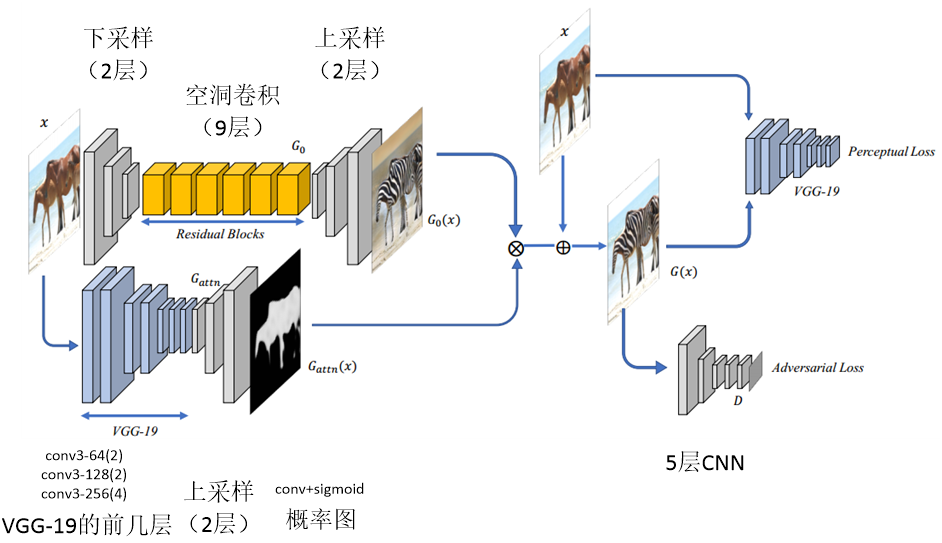https://www.cnblogs.com/darkknightzh/p/9333844.html$G(x)={{G}_{attn}}(x)\otimes {{G}_{0}}(x)+(1-{{G}_{attn}}(x))\otimes x$

${{G}_{attn}}(x)\otimes {{G}_{0}}(x)$代表前景区域，$(1-{{G}_{attn}}(x))\otimes x$代表背景区域。${{G}_{attn}}(x)$为前景区域的概率图，像素范围为[0, 1]。

${{L}_{D}}=\log (D(y))-\log (1-D(G(x)))$

${{L}_{G}}={{l}_{adv}}(G(x),y)+\lambda {{l}_{reg}}(x,G(x))$

${{l}_{adv}}(G(x),y)=-\log (-D(G(x)))$

${{l}_{reg}}(G(x),x)=\sum\limits_{l=1,2,3}{\frac{1}{{{H}_{l}}{{W}_{l}}}\sum\limits_{h,w}{(\left\| {{w}_{l}}\circ (\hat{F}(x)_{hw}^{l}-\hat{F}(G(x))_{hw}^{l}) \right\|_{2}^{2})}}$

${{l}_{reg}}$使用预训练的vgg-19网络的前三层加权得到。分别将输入图像x及生成的图像$G(x)$通过vgg-19网络前3层，得到对应的特征图，并计算特征图的l2 norm的平方，之后进行加权。各层权重经过大量实验得到为：

$({{w}_{1}},{{w}_{2}},{{w}_{3}})=(1/32,1/16,1/8)$